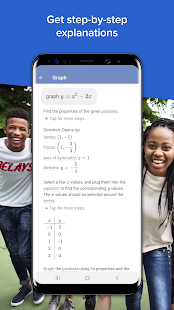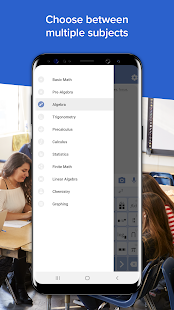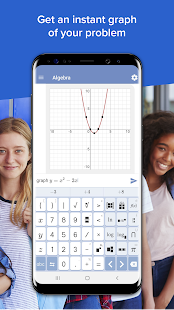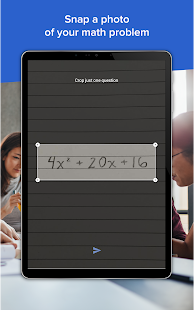Descriptions : Mathway is the world's smartest math calculator for algebra, graphics, calculus, and more! Just point your camera and snap a photo or type in your math homework question for step-by-step answers.
With millions of users and billions of problems solved, Mathway is like a private tutor at your fingertips, providing instant homework help anywhere, anytime.
Features :

+ Basic math / prealgebra (arithmetic, integers, fractions, decimals, roots, factors and more ...)
+ Algebra (linear equations / inequalities, quadratic equations / inequalities, absolute equations / inequalities, systems of equations, logarithms, functions, matrices, graphics and more ...)
+ Trigonometry / precalculus (trigonometric functions, identities, conic sections, vectors, matrices, complex numbers, sequences and series, and more ...)
+ Analysis (limits, derivatives, integrals and more ...)
+ Statistics (probability, permutations, combinations and more ...)

Required Android Versions : KitKat [4.4–4.4.4] - Lollipop [5.0–5.0.2] - Marshmallow [6.0 - 6.0.1] - Nougat [7.0 – 7.1.1] - Oreo [8.0-8.1]

## Download Mathway Scan Photos, Solve Problems v3.3.33 APK Free

com.bagatrix.mathway.android-254-v3.3.33.apk